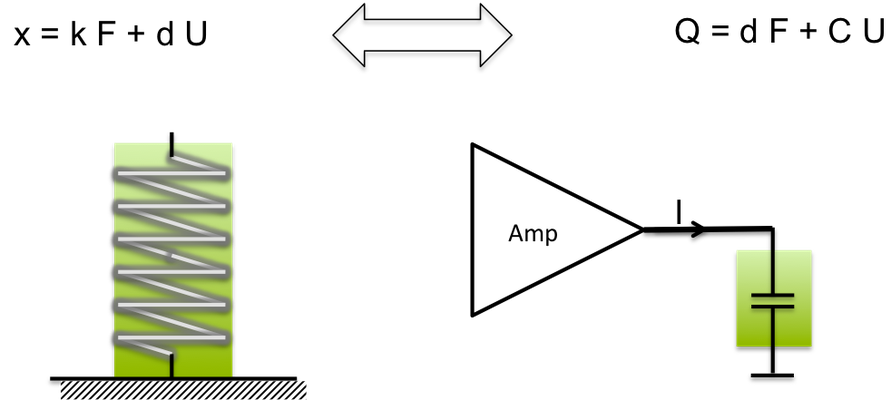# Electrical Behavior - Current during charging and de-charching

### Piezoelectric stack actuators are electrically capacitors. The piezo effect couples mechanical and electrical states:Dualism of piezo stack elements. Mechanical and electrical phenomena.

### To get an overview of the electrical power requirement to drive a piezo stack actuator, calculations with the assumption of an effective constant electrical capacity is in most cases sufficient:

The well-known equation for the current through a capacitor is

I = C d/dt U

For a sinus wave with amplitude U and frequency f, the amplitude of the current I flowing through the piezo stack is given by

I = 2 PI f C U

These formulas are helpful to select the right amplifier for a piezo stack. The influence of temperature and large signal is significant and thumbs of rules are needed to practically estimate current rating. The large signal behaviour of actuator –grade ceramics is non-linear and an increase of the current by a factor of two provides a good guess. Piezo actuators are electrical capacitors with superimposed effects related with of electro-mechanical coupling mechanism. Practically, the deformation of the piezo is linked with the superimposition of charges in the capacitor and the deformation rate (speed of displacement) is linked with an additional electrical current. Thus, the detailed analysis requires setting up and solving the equations with the couplings terms for the mechanics of piezo and loads.

## Piezo - Electrical Power Consumption

To select the right amplifier and to design the electrical supply, it is necessary to define the peak current need I peak and the average Pave. The current depend on the required maximum dynamic operation of the piezo and of course by the maximum voltage. The maximum speed of the displacement requires a maximum current Ip, which the amplifier has to supply. In the previous chapter the current has already been calculated for sine wave form operation. More generally, the as displacement of a piezo is in first approximation proportional to the voltage U

X = deff U

where deff is the effective factor for displacement of the piezo actuator in analogy to the piezoelectric constant for material. The speed of displacement V is proportional to the time derivative of the voltage

V = deff  d/dt U

The current flowing through a capacitor is proportional to time derivative of the voltage. The current, is proportional to the speed of displacement.

Example

The stack 5x5x20mm has a maximum displacement of 17 micrometer at 150 V.

The capacitance C of the stack is 1.4 µFarad.

When the piezo is driven to 150 V it takes a charge:
Q = 1.4
mF 150 V=1.4 10-6 As/V 150 V = 0,21 mAs.

The current needed to position the stack in 5 ms to the maximum length is:

I = 0,210 mAs/ 5 10-3s = 42 mA

The amplifier also need sufficient margin to consider the large-signal effect. The large signal effect describes the capacity for a large voltage swing. The nominal capacity is a value for small signals.

If higher dynamics is needed, e.g. driving the same piezo in 500 ms to 150V, a higher current is needed:

I = 0,210 mAs/ 500 10-6s = 420 mA

## Piezo Electrical Behavior – Power Loss

As in any electric component piezoelectric actuators causes some losses of electric energy. Usually, the calculation of the losses is carried out with a loss factor tan d or by an equivalent serial resistance (ESR).  In alternating current AC theorya dielectric loss factor is defined as:

tan delta = ESR 2 Pi f C

where tan delta is called loss tangent. For piezo actuators the loss tangent depends on the voltage amplitude. For small amplitudes, e.g. some Volts, the damping effect is quite small and the loss factor tan delta is typically 1% . For large signal operation, e.g. full amplitude swing from -30 to 150 V, the loss tangent is typically 10%. Square wave operation causes losses up to 20%. It is noted that for static operation (constant voltage or position) the losses are extremely small. This is a fundamental advantage against electromagnetic actuators which consumes significant power when maintaining a position.